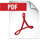# GC-TOFMS Application: Using Volcano Plots to Compare Vinyl Acetate Resin Samples Measured by Pyrolysis GC-MS

• Summary

## General

Advances in mass spectrometry are enabling analysis of micro samples and unknown components that were not observable before. As the volume of information acquired from mass spectrometry increases, researchers are calling for simple techniques to analyze the numerous components observed, and as a result, there is a rise in demand for comprehensive analytical techniques including multiple classification analysis.
In this work, we analyzed 2 samples in a high resolution GC-TOFMS, using EI, the most widely used ionization technique for GC-MS, and compared the data acquired by using a comprehensive analytical technique.

## Method

As model samples, 6 commercial vinyl acetate resins (adhesives) were used (sample A～F). A gas chromatography time-of-flight mass spectrometer (GC-TOFMS) was used for the measurements. Since emulsion samples including adhesives are difficult to analyze without preliminary treatment, the samples were subjected to pyrolysis. Also, a column shorter than a typical GC column was used along with a Fast GC temperature sequence to speed up the measurement process. The acquired data was examined using SpectralWorks Analyzer Pro, and 2 samples were compared by the Volcano Plot. Table 1 shows the pyrolysis fast GC/TOFMS measurement conditions.

## Table1. Measurement Conditions [Py-GC-TOFMS conditions]

System JMS-T200GC (JEOL) 600°C EI+: 70eV, 300μA DB-5msUI, 15m × 0.25mm, 0.25μm 50°C (1min)→30C° /min→330°C (1.7min) 300°C Split 100:1 1.0mL/min (Constant Flow) m/z 35-650 0.1 sec/spectrum AnalyzerPro（SpectralWork）

## Results

In MS Tips 243, same 6 vinyl acetate resin samples (A～F) were examined using PCA. Out of these, samples C and F, which had relatively similar TIC patterns, and samples A and C, which were not clearly separated by PCA (see Figure 3), were compared using the Volcano Plot. The Volcano Plot makes it possible to express individual components that constitute the differences between 2 samples, including reproducibility. Features of the Volcano Plot are as follows.## [Volcano Plot]

• The X axis represents the logarithm of the intensity ratio between the samples, while the Y axis represents the negative logarithm of the p value in variance analysis.
• Data points correspond to individual components.
• Points having larger values in the Y axis are identified as components having higher reproducibility and points having larger absolute values in the X axis as components having larger intensity ratios between the samples.
• In Figures 1 and 2, points that exceed 5% (p=0.05), a level generally considered significant, are shown in green.
• Squares (□) arranged in line on both sides of the figures represent the components observed only in respective samples.

Figure 1 shows TIC chromatograms and Volcano Plot results for samples C and F. While samples C and F share many components, sample F contains these shared components in larger quantities. The data shows that samples C and F, though made of similar ingredients, contain other components including additives in different quantities. Figure 2 shows TIC chromatograms and Volcano Plot results of samples A and C. Samples A and C have fewer shared components, featuring many components unique to each.

The Volcano Plot, using the results of variance analysis, made it possible to examine in detail the differences between the 2 samples. The characteristic components distinguishing the 2 samples were instantly identified by the intensity ratio (X) and reproducibility (Y). This demonstrates that the Volcano Plot is an effective tool for comparing 2 samples and extracting their characteristic components.## Figure 1. TIC chromatograms and Volcano Plot results of samples C and F## Figure 2. TIC chromatograms and Volcano Plot results of samples A and C## Figure 3. PCA scores of polyvinyl acetate samples